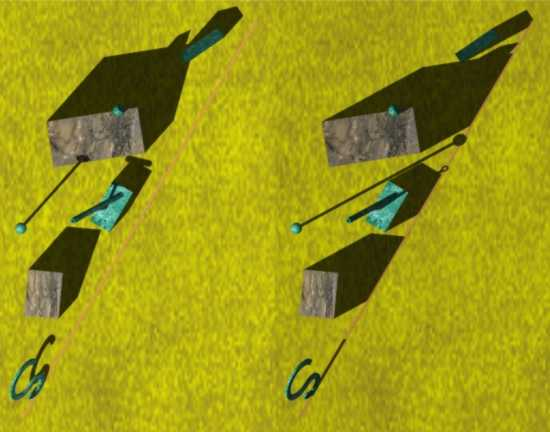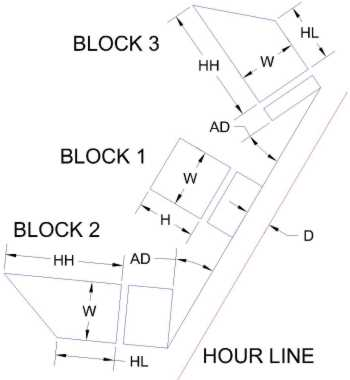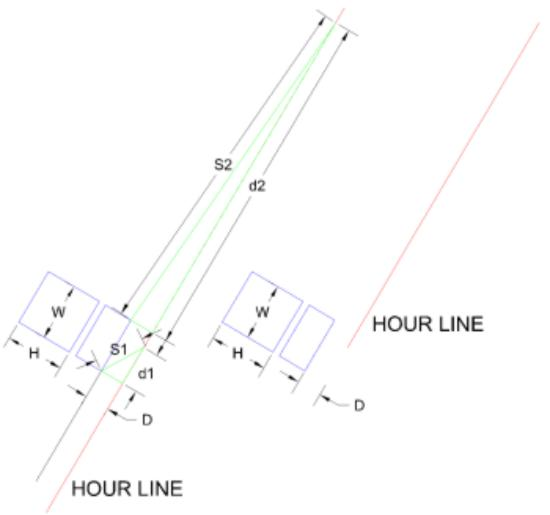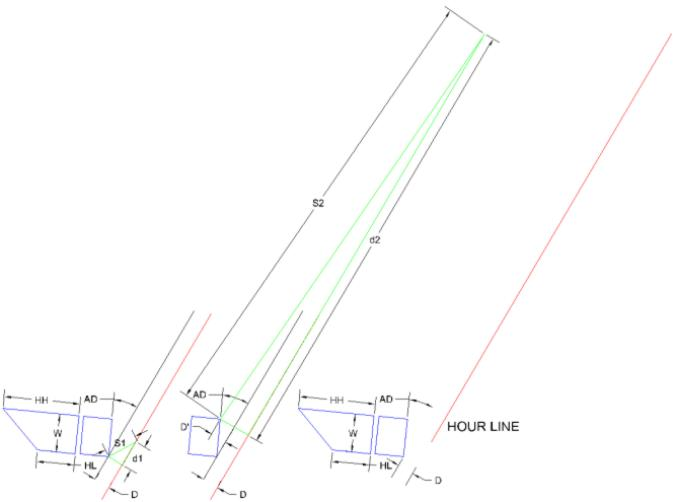The Sundial Primer created by Carl SabanskiThe Sundial Primer Index
 Shadow Plane Sundial Shadow Plane Sundial: a class of dial in which the gnomon is movable and is set by the observer so that it, and its shadow, lie in the sun's hour plane. The gnomon may be a plane, line or point. The dial plate can, if required, be any surface.Hour Plane: the plane which, at any instant, contains the sun, the observer and the north celestial pole. The style and the appropriate hour line lie in the hour plane. Style: the line in space which generates the shadow edge used to indicate the time on the dial plate. If a portion of an object lies in the sun's hour plane it will cast a shadow that indicates that particular moment in time. This allows you to create any number of shadow plane sundials that indicate particular times of the day. These sundials can be small or they can be monumental.Figure 1 illustrates a number of simple objects having some portion of that object positioned in the sun's hour plane.Figure 1: Shadow Plane Objects - A Plan View (CAD) Parts of all these objects are located on the sun's hour plane for 2:30 pm, local apparent time. The image on the left is taken at 1:30 pm and the one on the right at 2:30 pm on June 21. This plan view shows a number of blocks, a sphere, a cone, a hollow cylinder and a symbol. The shadows of all these objects will line up to indicate one particular time of day. However, not all these objects may be quite as they appear. Figure 2 shows these same objects from a slightly different angle.Figure 2: Shadow Plane Objects - A Different View (CAD)All the blocks are located in a slightly different position. However, every one of them has an edge located on the sun's hour plane. The very top of the sphere and the tip of the cone are also on the hour plane. The entire symbol at the left is located on the hour plane. The hole down the centre of the cylinder must not only be located on the hour plane but must also be at the same angle as the sun's altitude. If this is done, then at 2:30 pm on any day of the year all these objects, except for one, will align themselves along the hour line. On June 21, the shadows are their shortest and will grow until they reach their maximum length on December 21.The following information will explain how to calculate the hour plane angle relative to a horizontal plane and how to determine the distance a block must be displaced from the hour line. The hour line angles (X, HLA) for a horizontal sundial can be calculated as follows: X = arctan {sin ø * tan (h)} where ø is the latitude and h is the hour angle, in degrees, given by: h = (T24 - 12) * 15° and T24 is the time in 24-hour clock notation (hours after midnight) in decimal hours. The hour plane angle (HPA) can be calculated as follows: HPA = arctan (tan ø / sin X) Figure 3 illustrates a number of blocks that will be used as shadow plane objects. One is a rectangular block with a level top. The other two blocks have sloped tops and their dimensions are identical except for their thickness. The thickness does not matter. These are three of the blocks shown in the images above.Figure 3: Shadow Plane Objects Note the following: W(Block1) = W(Block2) = W(Block3) H(Block1) = HL(Block2) = HL(Block3) HH(Block2) = HH(Block3) AD(Block2) = AD(Block3) The distance (D) that the edge of the rectangular Block 1 is displaced from the hour line can calculated as follows: D = H / tan (HPA) This is the same distance for the side HL of Blocks 2 and 3. Block 2 and 3 have one end HH that is higher. These ends must be moved even further away so  the top edge of the block will lie in the hour plane. This distance can be calculated as shown above. The diagram also shows an angular displacement AD that the block must be turned off the hour line for alignment. This can be calculated as follows: Z = (HH - HL) / W AD = arcsin {Z / tan (HPA)} Click here to download a spreadsheet that will perform these calculations for you. Finally, the length of the hour line must be determined. Where does it start and end? Calculate the shadow lengths for the sides of the block on the days of the Summer and Winter Solstices or other appropriate day. This will give their minimum and maximum shadow lengths. Not all hours are available as the Winter Solstice nears. When the sun will no longer indicate a particular time depends upon the latitude. This must be established to find an appropriate date to determine the maximum shadow length for some hour lines. "The Dialist's Companion" can be used to speed up the process. An appropriate length for the hour line can now be determined. Let's take a look. The first example is for the uniform rectangular block. All the edges have the same height "H". The distance "D" is calculated as discussed above. Using "The Dialist's Companion" the altitude of the sun and the length of the shadow per unit height can be displayed for any date and time. In this case it is determined for 2:30 pm, local apparent time, on the Summer and Winter Solstices. The Winter Solstice is applicable case because the sun will be available to cast a shadow.  If only the sun's altitude was available the length of the shadow can be calculated as follows:Shadow Length = H / tan (Sun's Altitude)The shadow length "S1" will be shortest on the Summer Solstice and the shadow length "S2" will be longest on the Winter Solstice. Figure 4 illustrates how to determine the length of the hour line required to contain the entire shadow of the block throughout the year. This is done by associating "S1" with the front edge of the block and "S2" with the back edge.Figure 4: Shadow Plane Object Hour Line - Uniform Block On the Summer Solstice the shadow "S1" will start at the front edge and must terminate on the hour line. The distance "d1" can be calculated and defines the start of the hour line. d1 = SQRT ( S12 - D2)  where "SQRT" is the square root. On the Winter Solstice the shadow "S2" will start at the back edge and must terminate on the hour line. The distance "d2" can be calculated and defines the end of the hour line.d2 = SQRT ( S22 - D2)The shadow of the block will always fall within this range. A shorter hour line is possible by starting both "S1" and "S2" at the front edge. The result will be that the as the Winter Solstice is approached part of the block's shadow will start to move off the hour line until only the shadow of the front edge will fall on the line on the Winter Solstice. The second example is shown in Figure 5. The rectangular block is similar to the one in the first example except the back edge is higher.Figure 5: Shadow Plane Object Hour Line - Non-Uniform Block On the Summer Solstice the shadow "S1" will start at the front edge and must terminate on the hour line. The distance "d1" can be calculated and defines the start of the hour line. d1 = SQRT ( S12 - D2) On the Winter Solstice the shadow "S2" will start at the back edge and must terminate on the hour line. To calculate the distance "d2" the following distance is required:D" = D + D' = D + ( W x sin AD) The distance "d2" can  now be calculated and defines the end of the hour line.d2 = SQRT ( S22 - D"2) A shorter hour line is possible by starting both "S1" and "S2" at the front edge. In this case the distance "D" would be used to determine "S2" and calculate the value of "d2".  This would result in an hour line that is the same length as the one in the first example. As can be seen the hour lines can become quite long. Consider a design that covers the period between the equinoxes so the range of the hour line will be less. This would be very applicable if you live where you get snow  in the winter and can't use the sundial during that period. The position of the shadow cast by an edge can be determined for any day and any hour  the sun illuminates the block. You can have a lot of fun playing with shadow plane objects. Create a unique feature of what may appear to arbitrarily placed objects but that in reality will tell the time!